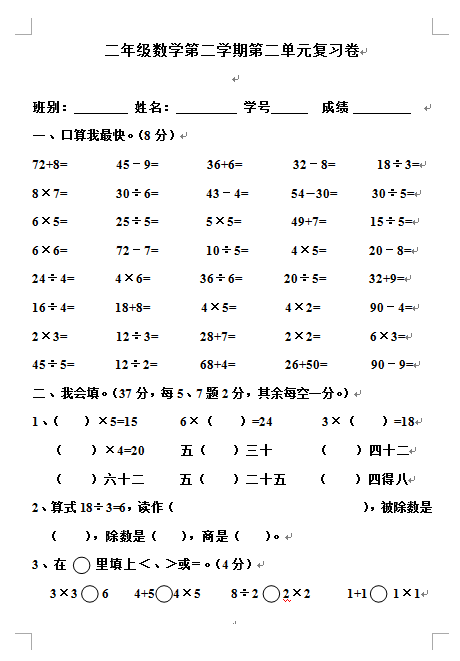72+8=        459=        36+6=        328=       18÷3=

8×7=        30÷6=        434=       5430=      30÷5=

【DOC文档5页】《小学二年级数学下册第二单元试卷》北师大版A4电子版资料_可直接打印_会员免费下载##### 评论信息